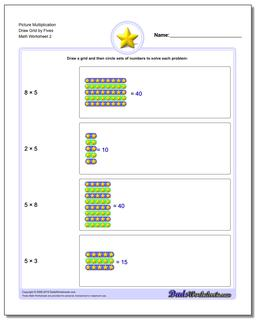# Math Worksheets: Picture Math Multiplication: Picture Math Multiplication: Picture Multiplication Draw Grid by Fives (Second Worksheet)## Picture Multiplication Draw Grid by Fives (Second Worksheet)

PropertyValue
DescriptionPicture Multiplication Draw Grid by Fives: Picture math multiplication worksheets where the student must draw the grid to solve the problem. (Second Worksheet)
Resource TypeWorksheet